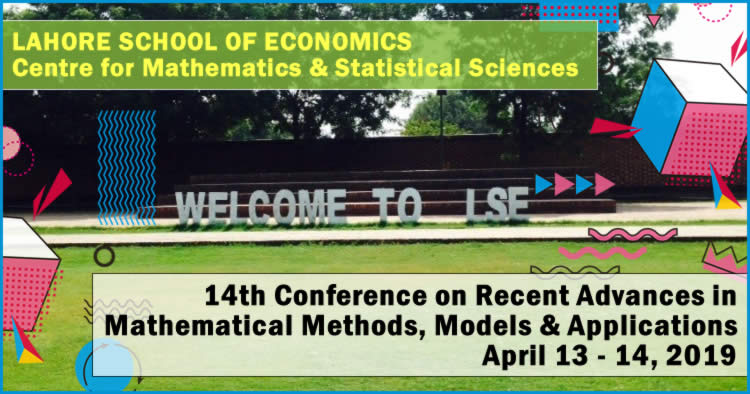# Recent Advances in Mathematical Methods, Models & Applications, LSC Lahore, Pakistan (April 13-14, 2019)New mathematical ideas may help in improving modeling of real problems, in deriving innovative and efficient numerical methods, and in developing approximate models which are amenable to mathematical analysis. There exists a non-trivial interplay between mathematics, mathematical modeling of complex systems and mathematical and computer methods oriented towards the qualitative and quantitative analysis of mathematical models. The goal of this conference is to bring together mathematicians and users of mathematics to talk to each other, to transfer problems, ideas and methods from one community to the other, so as to enhance progress in understanding. The “RAMMMA” tradition has these key features: the number of participants is usually bounded above by 70 with a mix of pure and applied interests; there are no parallel sessions so that all participants focus on each presentation and fully engage in each topic; and there is ample time for discussion of each presentation.

• Conference Name: 14th Conference on Recent Advances in Mathematical Methods, Models & Applications